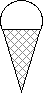EnchantedLearning.com is a user-supported site.
As a bonus, site members have access to a banner-ad-free version of the site, with print-friendly pages.

 You might also like: Divide Eight Birds Worksheet Printout Divide Fruit into Four Groups Worksheet Printout Divide Ten Pets Worksheet Printout Divide Fish by Five Worksheet Printout Divide Nine Flowers Worksheet Printout Today's featured page: Where I Live. Early Reader Book

 Go to the AnswersMath Pages EnchantedLearning.com Divide Six by 2, 3, and 6 Divide the items into groups to do division. Visual Division PagesDivision Pages

 Divide the balloons into two equal groups.How many balloons are there? How many groups of balloons are there? How many balloons are in each group?
6 ÷ 2 =

 Divide the gifts into three equal groups.How many gifts are there? How many groups of gifts are there? How many gifts are in each group?
6 ÷ 3 =

 Divide the ice cream cones into six equal groups.How many ice cream cones are there? How many groups of ice cream cones are there? How many ice cream cones are in each group?
6 ÷ 6 =

Enchanted Learning Search

 Search the Enchanted Learning website for: